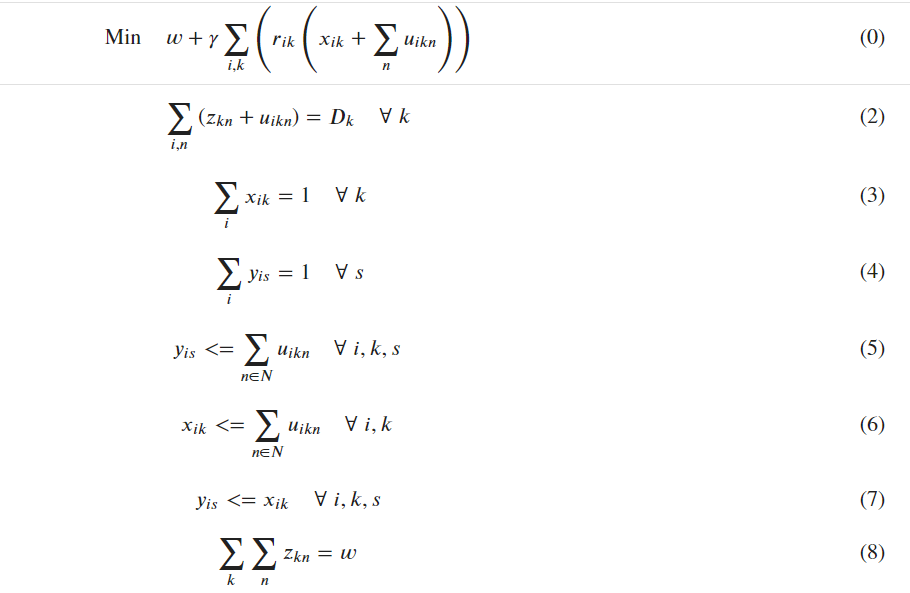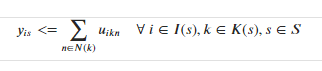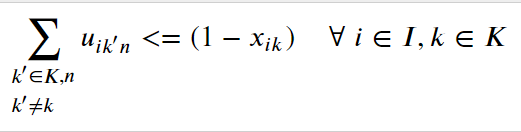•Gurobi Staff

Hi Vusal,

Here is how I would approach your first example.

Setup:

import gurobipy as gpfrom gurobipy import GRBimport gurobipy_pandas as gppdimport pandas as pdi = (1,2)k = (1,2,3)n = ("a", "b")s = ("c", "d")model = gp.Model()y = gppd.add_vars(model, pd.MultiIndex.from_product((i,s), names=("i", "s")), vtype=GRB.BINARY, name="y")u = gppd.add_vars(model, pd.MultiIndex.from_product((i,k,n), names=("i", "k", "n")), vtype=GRB.BINARY, name="u")

From here there are a few candidate approaches for aligning indices between the LHS and RHS of the constraint - either reindexing, merging Series/Dataframes, or perhaps the simplest: aligning

Reindexing doesn't seem to work in this case, merging will work but requires a little bit of work with indexes to retain the non-shared indexes (s,k) between the two operands of the merge.

The approach using pandas.Series.align starts by constructing the LHS and RHS:

LHS, RHS = y.align(u.groupby(["i", "k"]).sum())

LHS will look like this

i  s  k1  c  1    <gurobi.Var y[1,c]>      2    <gurobi.Var y[1,c]>      3    <gurobi.Var y[1,c]>   d  1    <gurobi.Var y[1,d]>      2    <gurobi.Var y[1,d]>      3    <gurobi.Var y[1,d]>2  c  1    <gurobi.Var y[2,c]>      2    <gurobi.Var y[2,c]>      3    <gurobi.Var y[2,c]>   d  1    <gurobi.Var y[2,d]>      2    <gurobi.Var y[2,d]>      3    <gurobi.Var y[2,d]>Name: y, dtype: object

and RHS will look like this

i  s  k1  c  1    u[1,1,a] + u[1,1,b]      2    u[1,2,a] + u[1,2,b]      3    u[1,3,a] + u[1,3,b]   d  1    u[1,1,a] + u[1,1,b]      2    u[1,2,a] + u[1,2,b]      3    u[1,3,a] + u[1,3,b]2  c  1    u[2,1,a] + u[2,1,b]      2    u[2,2,a] + u[2,2,b]      3    u[2,3,a] + u[2,3,b]   d  1    u[2,1,a] + u[2,1,b]      2    u[2,2,a] + u[2,2,b]      3    u[2,3,a] + u[2,3,b]Name: u, dtype: object

From here it is straightforward to construct the constraint

gppd.add_constrs(      model, LHS, GRB.LESS_EQUAL, RHS, name="con",)

Note that sometimes it may be easier to explicitly construct the index of the constraints first and individually align various elements in the expression, eg

constraint_index = pd.Series(    index=pd.MultiIndex.from_product([i,k,s], names=("i", "k", "s")))LHS, _ = y.align(constraint_index)RHS, _ = u.groupby(["i", "k"]).sum().align(constraint_index)gppd.add_constrs(    model, LHS, GRB.LESS_EQUAL, RHS, name="con",)

You can find a few more examples by searching posts here with the gurobipy-pandas tag.

- Riley

•Thanks, Riley. This is indeed a cleaner solution. This is the math programming I am trying to implement. As you can see, I have several misaligned constraints.However, when I try the constraint 5 on actual index set values using the solution you provided, I am getting the following error:

ValueError: lhs series has missing values
df = df.reset_index(drop=True).set_index(['i', 's'])y = gppd.add_vars(model, df, vtype=GRB.BINARY, name='string_assignment')
df = df.reset_index(drop=True).set_index(['i', 'k', 'n'])u = gppd.add_vars(model, df, vtype=GRB.BINARY, name='asset_count')
LHS, RHS = y.align(u.groupby(["i", "k"]).sum())cons = gppd.add_constrs(model, LHS, GRB.LESS_EQUAL, RHS, name="con")

Where might things have gone wrong?  Is the approach same for the constraints 6 and 7? Thanks!

•Gurobi Staff

Hi Vusal,

I will need to be able to reproduce the error in order to help.

Are you able to make df_string and df_asset_counts available as CSV files via some cloud based storage such as Google Drive?

- Riley

•That would be very difficult for me to share. But here are a few tips that perhaps can help. LHS and RHS have the same number of - 12,427 - rows. When I print the difference, the indices are the same - there are no missing values in resource_id, string_id and store_num.

 LHS, RHS = y.align(u.groupby(["i", "k"]).sum()) idx1 = LHS.index idx2 = RHS.index print (idx1.difference(idx2))Output:MultiIndex([], names=['i', 's', 'k'])
•Gurobi Staff

Hi Vusal,

Can you run pandas.show_versions() and paste the result?

- Riley

•INSTALLED VERSIONS------------------python           : 3.9.9.final.0pandas           : 2.0.0numpy            : 1.24.2pytz             : 2023.3dateutil         : 2.8.2setuptools       : 67.6.1pip              : 23.1.2jinja2           : 3.1.2pyarrow          : 12.0.0tabulate         : 0.9.0tzdata           : 2023.3
•Gurobi Staff

Can you check that the set of values for "resource_id" in the index for u and y are the same? The get_level_values method on the index will help.

Can you also print u.Index.dtypes (and the same for y)?

•Below are the dtypes for y_is and u_ikn

i     int64s     objectdtype: objecti   int64k   int64n   int64dtype: object
•You're right. The indices don't match, as seen below - the first is a subset of the other.

Index([1, 3, 8, 2, 4, 6, 16, 9, 14], dtype='int64', name='i')Index([4, 2, 5, 3, 10, 1, 15, 11, 6, 8, 12, 7, 9, 16, 13, 14], dtype='int64', name='i')

i for y is correct (the first list above). I need to use the same i for u_ikn. How would you suggest to fix this without defining a new u_ikn because I need u_ikn with the second index set above as well in my model. For me these two sets of indices come from different pandas series.

•Gurobi Staff

When you call align, it will introduce null values in LHS for those resource_id values that are in u but not y. If you filter LHS and RHS using LHS.notna() then this should solve the problem. Alternatively filter u by the y resource_id values before doing the groupby and sum.

•Gurobi Staff

Perhaps the "join” parameter of Series.align could also be used to solve the problem but I'm not currently able to test it.

•Thank you very much. Adding LHS.notna() and RHS.notna() seems to solve the issue.

LHS, RHS = y.align(u.groupby(["i", "k"]).sum(), join='left' or 'inner') also seems to work, but I need to do a bit more QA.

How would you approach constraints 6 and 7? The below attempts using align were not successful:

LHS, RHS = x.align(u.groupby(["i", "k"]).sum())constulant_changeover_coverage =  gppd.add_constrs(model, LHS.notna(), GRB.LESS_EQUAL, RHS.notna(), name="consultant_string_coverage")LHS, RHS = y.align(x)cons = gppd.add_constrs(model, LHS.notna(), GRB.LESS_EQUAL, RHS.notna(), name="con")string_store_coverage =  gppd.add_constrs(model, y, GRB.LESS_EQUAL, x, name="string_store_coverage")
•Gurobi Staff

Hi Vusal,

Constraints 6 and 7 should follow the same approach.  Your code above doesn't quite reflect what I was imagining though.  Note that LHS.notna() will be a Series of booleans - not something we want as an argument to add_contrs.  Let's set up an example where both LHS and RHS have elements in the common index that the other doesnt:

i_1 = (1,2,4)i_2 = (1,3,4)k = (1,2,3)n = ("a", "b")s = ("c", "d")model = gp.Model()y = gppd.add_vars(model, pd.MultiIndex.from_product((i_1,s), names=("i", "s")), vtype=GRB.BINARY, name="y")u = gppd.add_vars(model, pd.MultiIndex.from_product((i_2,k,n), names=("i", "k", "n")), vtype=GRB.BINARY, name="u")

There are several ways of defining an appropriate LHS and RHS:

LHS, RHS = y.align(u.groupby(["i", "k"]).sum())filter = LHS.notna() & RHS.notna()LHS, RHS = LHS[filter], RHS[filter]

or

LHS, RHS = y.align(u.groupby(["i", "k"]).sum(), join="inner")

or

i = y.index.get_level_values("i").intersection(u.index.get_level_values("i"))constraint_index = pd.Series(    index=pd.MultiIndex.from_product([i,k,s], names=("i", "k", "s")))LHS, _ = y.align(constraint_index, join="inner")RHS, _ = u.groupby(["i", "k"]).sum().align(constraint_index, join="inner")

then we can add as usual.

gppd.add_constrs(      model, LHS, GRB.LESS_EQUAL, RHS, name="con",)

- Riley

•Hi Riley:

While going through the LP file, I noticed a missing detail in the formulation of the constraint where index k is dependent on index s. Sorry about this mistake on my end.At the moment, I am reading (i,s) pairs from one df and (i,k,n) triplets from another data frame which I use to create y and u variables, respectively, first and then create the constraint using the index alignment as you suggested above.

LHS, RHS = y.align(u.groupby(["i", "k"]).sum(), join="inner")

The variable definition is fine, but what would you suggest dealing with a dependent index set, and how would you design a pandas data frame to model these constraints?

•Gurobi Staff

Hi Vusal,

In this case I would use the alternative approach discussed above where you construct the index first, and then align LHS and RHS to the index. How you construct the index will depend on how I(s) and K(s) is defined.

For example if you had functions

def index_I(s):    ...     return ... def index_K(s):    ...    return ...

Then you can use the following to construct a Series with required index

constraint_index = pd.Series(    index=pd.MultiIndex.from_tuples(        [(i,k,s) for s in S for i in index_I(s) for k in index_K(s)],        names=("i", "k", "s")    ))

You may need to filter with the following

filter = LHS.notna() & RHS.notna()LHS, RHS = LHS[filter], RHS[filter]

after aligning LHS and RHS to constraint_index.

- Riley

•Hi Riley:

I start as follows:

df= df_joined[['i', 's', 'k']].head()constraint_index = pd.Series(index = pd.MultiIndex.from_frame(df, names= ['i', 's', 'k']))

Then, I do the following:

LHS, _ = y.align(constraint_index, join="inner")RHS, _ = u.groupby(["i", "k"]).sum().align(constraint_index, join = "inner")con = gppd.add_constrs( model, LHS, GRB.LESS_EQUAL, RHS, name="con")print (con)

When I run above I am getting the

KeyError: 'series must be aligned'

When I print LHS and RHS, I get the following. To me, they are aligned since the indices are common on both sides. Any idea what's the problem here? Is it the order of columns in the series? If so, how can I fix it?

i             s          k1             22D        2            <gurobi.Var y[1,22D]>                        468           <gurobi.Var y[1,22D]>2             22F       272           <gurobi.Var y[2,22F]>                        406           <gurobi.Var y[2,22F]>                        22            <gurobi.Var y[2,22F]>Name: y, dtype: objecti            k          s2            272        22F          u[2,272,2022_14] + u[2,272,2022_12] + ...             406        22F          u[2,406,2022_11] + u[2,406,2022_10] + ...             22         22F          u[2,22,2022_05] + u[2,22,2022_04] + ..1            2          22D          u[1,2,2022_17] + u[1,2,2022_14] + ...             468        22D          u[1,468,2022_13] + u[1,468,2022_15] ...Name: u, dtype: object

Also, it seems I don't need this filter since there is no NAs, but when I run, I get the following error.

filter = LHS.notna() & RHS.notna()
LHS, RHS = LHS[filter], RHS[filter]
pandas.errors.IndexingError: Unalignable boolean Series provided as indexer (index of the boolean Series and of the indexed object do not match).
•Gurobi Staff

Hi Vusal,

Any idea what's the problem here? Is it the order of columns in the series?

Yes that seems to be the problem.  We can fix this by adding these lines after aligning:

LHS = LHS.reorder_levels(constraint_index.index.names)RHS = RHS.reorder_levels(constraint_index.index.names)

After this the filtering should work too, but as you said it is not needed in this instance.

- Riley

•Thanks very much!

•Hi Riley:

I have a following constraint which I am trying to implement.I am having a problem with aligning the indices. What would be the right approach to align LHS and RHS here? I am a bit confused. X and U are binary assignment variables defined same as above on index i and k and i, k and n respectively

•For example, below is a filtered Pandas df for one set of i, k', k, n after data manipulation and wrangling.

       i          k'              k             n  0      1          287            32          2023-071      1          110            32          2023-072      1          172            32          2023-073      1          137            32          2023-07
•Gurobi Staff

Hi Vusal,

There's at least a couple of approaches you can use.  I'll use the following example

import gurobipy as gpfrom gurobipy import GRBimport gurobipy_pandas as gppdimport pandas as pdi = (1,2)k = (1,2,3)n = ("a", "b")model = gp.Model()x = gppd.add_vars(model, pd.MultiIndex.from_product((i,k), names=("i", "k")), vtype="B")u = gppd.add_vars(model, pd.MultiIndex.from_product((i,k,n), names=("i", "k", "n")), vtype="B")

Approach 1

This involves manually constructing the value of the LHS for each i,k, by mapping the index of x (assuming this has the right i,k values) to a function.  In this approach I use a dataframe for u as the filtering is more readable but you can define a similar approach with the u series using u.index.get_level_values.

u_df = u.reset_index()def make_lhs(ind):  # the LHS for each i,k    i,k = ind    return u_df.query("i == @i and k != @k")["u"].sum()gppd.add_constrs(    model,    x.index.map(make_lhs),    GRB.LESS_EQUAL,    1 - x,    name="con",)

Approach 2

This approach recognizes that for a particular i, k we have

$\sum_{k' \in K, n: k' \neq k}u_{ik'n} = \sum_{k' \in K, n}u_{ik'n} - \sum_{n}u_{ikn}$

Both sums on the RHS can be calculated using a standard gurobipy-pandas workflow

sum_u_i = u.groupby("i").sum()  # first sumsum_u_i_k = u.groupby(["i", "k"]).sum()  #second sumgppd.add_constrs(model, sum_u_i - sum_u_i_k, GRB.LESS_EQUAL, 1 - x)

This approach is conciser and runs faster, although may not always be applied more generally to other scenarios.

- Riley

•Hi Riley

Thanks for the approaches. Variable definition is fine for i, k and n but constrain is applied to certain subset of i,k and n.

To clarify, the index i is the same on both sides of the constraint. The relationship between k and k' is not straightforward - it's not simply k' != k. For each k, there is a set of k' index values (where k! = k') that constraint is applied. I think an approach where we define the constraint indices first and then implement the LHS and RHS will probably work better.

Vusal

•Gurobi Staff

Hi Vusal,

Ok, let's try using your dataframe and changing the setup accordingly.

Setup:

import gurobipy as gpfrom gurobipy import GRBimport gurobipy_pandas as gppdimport pandas as pdi = (1,2)k = (32,287,110,172,137)n = ("a", "b")model = gp.Model()x = gppd.add_vars(model, pd.MultiIndex.from_product((i,k), names=("i", "k")), vtype="B")u = gppd.add_vars(model, pd.MultiIndex.from_product((i,k,n), names=("i", "k", "n")), vtype="B")u_df = u.reset_index().rename(columns={"k":"k'", 0:"u"})df = pd.DataFrame({    "i":[1,1,1,1],    "k'":[287, 110, 172, 137],    "k":[32,32,32,32],    "n":["a", "b", "a", "a"],})

u_df has columns i, k', n and u.  The column u has the variables associated with i, k', n.  The column k' is the same as k, just renamed.

Solution:

LHS = (    pd.merge(df, u_df, on=["i", "k'", "n"], how="left")  # has columns, i, k', k, n, u    .groupby(["i", "k"])["u"].sum())# LHS has columns i,k and is the corresponding LHS for that index pairgppd.add_constrs(    model,    LHS,    GRB.LESS_EQUAL,    1 - x,)

If x contains (i,k) indices your LHS doesn't (because they weren't in the df dataframe) then you'll have to align first.  Does this work?

- Riley